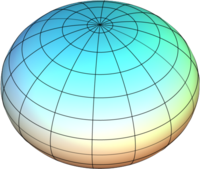# Angular eccentricity

﻿
Angular eccentricity

In the study of ellipses and related geometry, various parameters in the distortion of a circle into an ellipse are identified and employed: Aspect ratio, flattening and eccentricity. All of these parameters are ultimately trigonometric functions of the ellipse's modular angle, or angular eccentricity, the conventional denotation being the Greek letter "alpha",$\alpha\,\!$.

## Definition

The angular eccentricity of an ellipse with semi-major axis, or transverse radius,$a\,\!$, and semi-minor axis, or conjugate radius,$b\,\!$, is defined as$\alpha=\arccos\left(\frac{b}{a}\right)\,\!$

## Elliptic parameters

In the following, the previously defined ellipse with radii$a\,\!$ and$b\,\!$ will be utilized.

### Aspect ratio

The most tangible characteristic of an ellipse is the diametric quotient of the minor axis to the major axis, the aspect ratio.

name value in terms of$a\,\!$ and$b\,\!$ value in terms of$\alpha\,\!$
aspect ratio$\frac{b}{a}\,\!$$\cos(\alpha)\,\!$

### Eccentricity

The eccentricity is actually a trio of factors: The primary, or first, eccentricity,${\color{white}_.}\varepsilon{\color{white}^.}\,\!$, (or just "${\color{white}_.}e{\color{white}^.}\,\!$"), the second eccentricity,$\varepsilon'\,\!$, and the third eccentricity,$\varepsilon''\,\!$ or$\sqrt{m}\,\!$.

symbol value in terms of$a\,\!$ and$b\,\!$ value in terms of$\alpha\,\!$$\varepsilon^2\,\!$$\frac{a^2-b^2}{a^2}\,\!$$\sin^2(\alpha)\,\!$${\varepsilon'}^2\,\!$$\frac{a^2-b^2}{b^2}\,\!$$\tan^2(\alpha)\,\!$${\varepsilon''}^2=m\,\!$$\frac{a^2-b^2}{a^2+b^2}\,\!$$\frac{\sin^2(\alpha)}{2-\sin^2(\alpha)}\,\!$

Since they are mostly used in that form anyway, the eccentricities are usually found and kept in their squared form.

The primary eccentricity could be regarded as the complementary aspect ratio, as it is the ratio of the linear eccentricity to the semi-major axis:$\varepsilon=\frac{c}{a}\,\!$

### Flattening

The flattening, or ellipticity, in contrast, is self-explanatory, as it defines the degree of "squashing", from no flattening (a perfect circle) to complete flattening (a straight line).

symbol value in terms of$a\,\!$ and$b\,\!$ value in terms of$\alpha\,\!$$f\,\!$$\frac{a-b}{a}\,\!$$2\sin^2\left(\frac{\alpha}{2}\right)\,\!$$f'\,\!$$\frac{a-b}{b}\,\!$$\frac{2\sin^2(\frac{\alpha}{2})}{1-2\sin^2(\frac{\alpha}{2})}\,\!$$f''=n\,\!$$\frac{a-b}{a+b}\,\!$$\tan^2\left(\frac{\alpha}{2}\right)\,\!$

While the aspect ratio would seem to be the ideal parameter to find an unknown axis (usually$b\,\!$), it is usually the inverse (primary) flattening that is provided:$\begin{matrix}\mathrm{E.g.,\;\;}a=6378,\;\frac{1}{f}=300\!:\;b=a(1-\frac{1}{300})=a\cos(\alpha)=6356.74\;\\\end{matrix}\,\!$

## Oblate vs. prolate

If an ellipse is rotated about one of its axes, it sweeps an ellipsoid of revolution. Rotation about the major axis produces a prolate spheroid, or prolatum; rotation about the minor axis produces an oblate spheroid, or oblatum.← Oblate;   Prolate →Rotation causes most large celestial bodies – stars, planets and large satellites – to form oblate spheroids. Planetodetic formulation therefore applies the oblate format, which follows standard elliptic parametrization.

## Applications

For the most part, elliptic formularies ignore the angular eccentricity for the more familiar and notationally concise$\varepsilon^2\,\!$,$\varepsilon'^2\,\!$, and${\color{white}_.}f{\color{white}^.}\,\!$. However, these parameters don't provide the clear and obvious transformational relationships and structure. Consider the basic elliptic integrand at point P:$\begin{matrix}{}_{\color{white}.}\\ \sqrt{1-\sin^2(P)\varepsilon^2}\!&=&\!\!\sqrt{1-(\sin(P)\sin(\alpha))^2},\qquad\qquad\qquad\qquad\qquad\qquad\\ &&\!\!\!\!\!\!{}_{=\;\sqrt{1-(1-\cos^2(P))\sin^2(\alpha)},}\qquad\qquad\qquad\qquad\qquad\\\\ &=&\!\!\!\!\!\sqrt{\cos^2(\alpha)+(\cos(P)\sin(\alpha))^2},\qquad\qquad\qquad\qquad\\ &&{}_{\;=\;\cos(\alpha)\sqrt{(\cos(P)\tan(\alpha))^2+1},}\qquad\qquad\qquad\qquad\qquad\\\\ &=&\!\!\!\!\!\!\!\!\!\!\!\!\sqrt{\cos^2(\alpha)+\frac{1}{2}(1+\cos(2P))\sin^2(\alpha)},\qquad\qquad\\ &=&\!\!\!\!\!\!\sqrt{\cos^2(\alpha)+\frac{1}{2}\sin^2(\alpha)+\frac{1}{2}\cos(2P)\sin^2(\alpha)},\qquad\\ &&\!\!\!\!\!\!\!\!\!\!\!\!\!\!\!{}_{=\sqrt{1-\frac{1}{2}e^2+\frac{1}{2}\cos(2P)e^2},}\qquad\qquad\qquad\qquad\qquad\\\\ &=&\!\!\!\sqrt{\cos^4\left(\frac{\alpha}{2}\right)+\sin^4\left(\frac{\alpha}{2}\right)+2\cos(2P)\sin^2\left(\frac{\alpha}{2}\right)\cos^2\left(\frac{\alpha}{2}\right)},\\\\ &=&\!\!\!\!\!\!\cos^2\left(\frac{\alpha}{2}\right)\sqrt{1+2\cos(2P)\tan^2\left(\frac{\alpha}{2}\right)+\tan^4\left(\frac{\alpha}{2}\right)},\qquad\\\\ &=&\!\!\!\!\sqrt{\frac{1}{(1+n)^2}+\frac{1}{2}\cos(2P)n^2+\frac{1}{4}n^2}\;=\;\frac{\sqrt{1+2\cos(2P)n+n^2}}{1+n}. \\{}^{\color{white}.}\end{matrix}\,\!$

While one may consider such ability to convert as just gratuitously frivolous, there is at least one valid reason, as the Binomial series expansion (which planetodetic formularies frequently use) for${}^{\sqrt{1+2\cos(2P)\tan^2\left(\frac{\alpha}{2}\right)+\tan^4\left(\frac{\alpha}{2}\right)}}\,\!$ converges a lot quicker than the one for${}^{\sqrt{1-(\sin(P)\sin(\alpha))^2}}\,\!$ which, in turn, converges quicker than${}_{\sqrt{(\cos(P)\tan(\alpha))^2+1}}\,\!$'s (which—in line with basic, series expansion theory—doesn't even converge when${}^{(\cos(P)\tan(\alpha))^2}\,\!$ ≥ 1). Friedrich Bessel firstly used the notation "n" in 1837 on calculation of meridian arc length.

Furthermore, as${}_{\cos^{\frac{1}{2}}(\alpha)\approx\;\cos^2\left(\frac{\alpha}{2}\right)\approx\;\cos^8\left(\frac{\alpha}{4}\right)\approx\;\cos^{\!\!\frac{4^{{}^{q}}}{2}}\left(\frac{\alpha}{2^q}\right)}\,\!$, there are likely other, even more efficient, series expansions possible (if not even efficiently practical approximations to a general transcendental elliptic integral).
Another example is the equation for authalic surface area:$\begin{matrix}{}_{\color{white}.}\\{}^{\color{white}\cdot}\mathrm{Oblate}\!\!\!&=&\!\!\!\!\!\!\!2\pi\left(a^2+\frac{b^2}{e}\ln\left(\frac{\sqrt{1-\varepsilon^2}}{1-\varepsilon}\right)\right),\qquad\qquad\quad\\\\ &=&\!\!\!2\pi\left(a^2+b^2\csc(\alpha)\ln\left(\cot\left(\frac{90^\circ-\alpha}{2}\right)\right)\right);\\{}^{\color{white}.}\end{matrix}\,\!$$\begin{matrix}{}_{\color{white}.}\\\mathrm{Prolate}\!\!\!&=&\!\!\!2\pi\left[ab\frac{\arcsin(\varepsilon)}{\varepsilon}+b^2\right]=2\pi\left[a^2\frac{\alpha}{\sin(\alpha)\cos(\alpha)}+b^2\right],\\\\ &=&\!\!\!\!\!\!\!\!2\pi\left[a^2\frac{2\alpha}{\sin(2\alpha)}+b^2\right]=2\pi\left[\frac{a^2}{\operatorname{sin\!c}(2\alpha)}+b^2\right].\qquad\\{}^{\color{white}.}\end{matrix}\,\!$

While one certainly can use$\varepsilon\,\!$ to define and express this type of equation, using$\alpha\,\!$ frequently provides a more illustrative—if not even its definitively mathematical—origin.

Wikimedia Foundation. 2010.

### Look at other dictionaries:

• Eccentricity — may refer to: * Eccentricity (behavior), unusual or odd behavior on the part of a person, as opposed to being normal * Eccentricity (mathematics), a parameter associated with every conic section * Eccentricity vector * Eccentricity (graph theory) …   Wikipedia

• Eccentricity vector — In astrodynamics, the eccentricity vector of a conic section orbit is the vector pointing towards the periapsis and with magnitude equal to the orbit s scalar eccentricity. The magnitude is unitless. CalculationThe eccentricity vector mathbf{e} …   Wikipedia

• Orbital eccentricity — This article is about eccentricity in astrodynamics. For other uses, see Eccentricity (disambiguation). An elliptic Kepler orbit with an eccentricity of 0.7 (red), a parabolic Kepler orbit (green) and a hyperbolic Kepler orbit with an… …   Wikipedia

• Ellipse — Elliptical redirects here. For the exercise machine, see Elliptical trainer. This article is about the geometric figure. For other uses, see Ellipse (disambiguation). Not to be confused with ellipsis. An ellipse obtained as the intersection of a… …   Wikipedia

• Longitude — For Dava Sobel s book about John Harrison, see Longitude (book). For the adaptation of Sobel s book, see Longitude (TV series). Map of Earth Longitude (λ) Lines of longitude appear vertical with varying curvature in this projection, but are… …   Wikipedia

• Latitude — This article is about the geographical reference system. For other uses, see Latitude (disambiguation). Map of Earth Longitude (λ) Lines of longitude appear vertical with varying curvature in this projection, but are actually halves of great… …   Wikipedia

• List of mathematics articles (A) — NOTOC A A Beautiful Mind A Beautiful Mind (book) A Beautiful Mind (film) A Brief History of Time (film) A Course of Pure Mathematics A curious identity involving binomial coefficients A derivation of the discrete Fourier transform A equivalence A …   Wikipedia

• List of gravitationally rounded objects of the Solar System — Objects in the Solar System By orbit By size By discovery date …   Wikipedia

• Spheroid — A spheroid is a quadric surfaceobtained by rotating an ellipse about one of its principal axes; in other words, an ellipsoid with two equal semi diameters. If the ellipse is rotated about its major axis, the result is a prolate (elongated)… …   Wikipedia

• Radius of curvature (applications) — The distance from the center of a sphere or ellipsoid to its surface is its radius. The equivalent surface radius that is described by radial distances at points along the body s surface is its radius of curvature (more formally, the radius of… …   Wikipedia